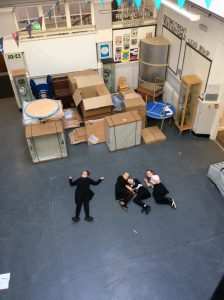# Making Maths Marks

Some of the P6 pupils have been learning to calculate area, using the formula: area = length x breadth, and also to add up all the edges to calculate perimeter.

Here they are working in teams to measure the area with metre sticks and marking the length and breadth on the floor: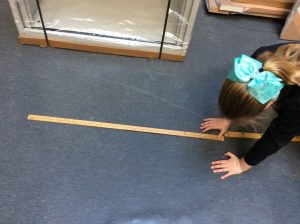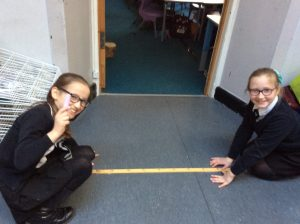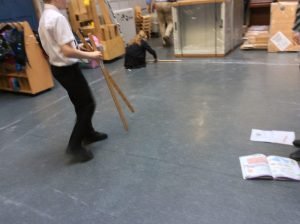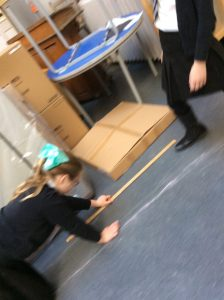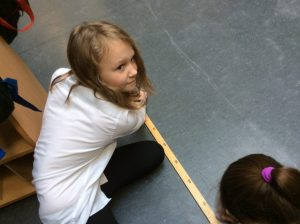Some posing pics: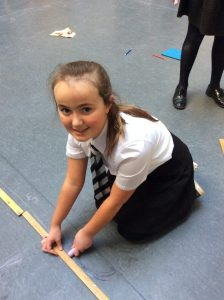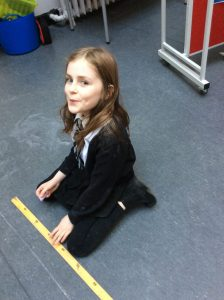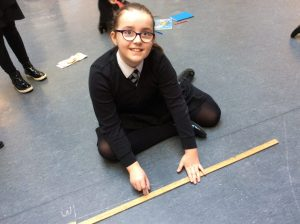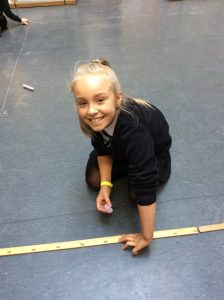Measuring is hard work: